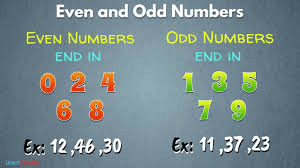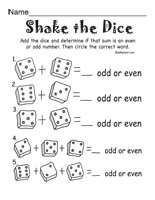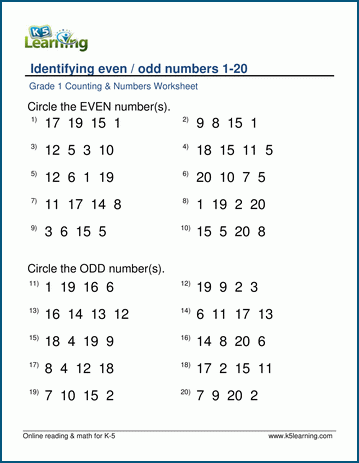# ODD AND EVEN NUMBER

HELLO CHILDREN, WELCOME BACK TO CLASS

Even number is a number that can be divided into two equal groups.

An odd number is a number that cannot be divided into two equal groups.

Even numbers end in 2, 4, 6, 8 and 0 regardless of how many digits they have (we know the number 8844 because it ends with 4.

Odd numbers end in 1, 3, 5, 7, 9.

We can also say that an even number is a number that can be divided exactly by 2 while an odd number cannot be divided exactly by 2 as there will be remainder.Adding and Subtracting Even and Odd Numbers

When we add (or subtract) odd or even numbers the results are always:

Even + Even = Even    e.g.   2 + 4 = 6

Even + Odd =   Odd      e.g.  6 + 3 = 9

Odd + Even =   Odd       e.g.  5 + 12 = 17

Odd + Odd =    Even      e.g.  3 + 5 = 8

Multiplying Even and Odd Numbers

When we multiply odd or even numbers the results are always:

Even × Even = Even   e.g.  4 × 8 = 32

Even × Odd = Even     e.g.   4 × 7 = 28

Odd × Even = Even      e.g.   5 × 8 = 40

Odd × Odd =    Odd       e.g.    5 × 7 = 35

ActivityYour Opinion Matters! Quickly tell us how to improve your Learning Experience HERE